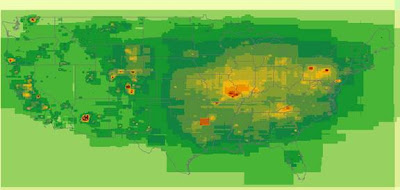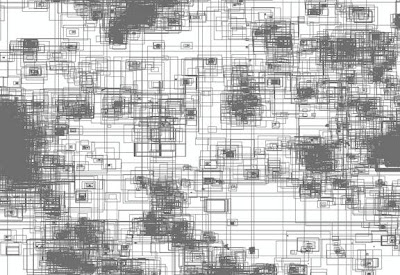## October 27, 2009

### WMS requests intensity map

A nifty way of visualizing requests to a Web Map Service is to analyse the server logs. My server of choice is Apache, and all WMS requests recorded in the Apache access log contain a bounding box. Using Perl, I parsed the log and extracted the coordinates of the bounding boxes matching on the string 'bbox'. Using PDL, each defined rectangle added another pixel to a blank 16-bit TIFF. The result looks like this, after using a color ramp in ArcMAP:Before I settled on the method described in this post, I tried a few other ways of visualizing the intensity of WMS requests. One other method inserted the bounding boxes into a Postgres table, and output a shapefile. Those shapefiles were quite large and difficult to handle, though they did make some interesting pictures.Now, a few details about the process I found worked best.

A matrix in PDL is called a 'piddle.'

PDL requires the netpbm binaries to be installed, and the PATH variable should contain a pointer to their installation folder.

PDL might give this error: “Error reading magic number from Netpbm image stream.” But you can ignore it because the image is still produced.

PDL normally outputs 8-bit TIFF files. A TIFF with only 8-bits is not enough to contain all the hits that every pixel receives because the maximum value of any pixel in an 8-bit image is 255. I had to output 16-bit TIFF, for which the maximum value is 65,535.

Load the required modules, and initialize some variables. This script works against a service in geographic projection, but it could be modified to work on any projection. Left, right, top and bottom define the crop region of the map. This reduces processing time and memory requirements if we ignore a lot of the Pacific and anything North of 50 degrees latitude. Columns and rows or the TIFF are computed from the bounds and from the degree size. Degree size determines the final resolution of the TIFF. A degree of size 8 will make pixels the same size as 7.5 minute quadrangles. The larger the number, the longer this script will run and the larger the output files.

`use PDL;use PDL::NiceSlice;use PDL::IO::Pic;use PDL::IO::FlexRaw;my \$degree_size = 128;my \$myfile = "log";my \$left = -125;my \$right = -65;my \$top= 50;my \$bottom = 23;my \$width = \$right-\$left;my \$height = \$top - \$bottom;my \$cols = \$width * \$degree_size;my \$rows = \$height * \$degree_size;my \$blank_slate = zeroes(\$cols,\$rows)->ushort;open(LOG, "log.txt") || die;`

Using a while(<LOG>) loop, go through every line of the log and using a regular expression, find the four coordinates. Replace the percent encoded characters, and split at the comma.

`#for each line of the logif (m/REQUEST=GETMAP/i) { my (\$bboxString) = (\$_ =~ m/BBOX=(.+?)[\&|\n|\s]/i); \$bboxString =~ s/%2C/,/g; \$bboxString =~ s/%2D/-/g; \$bboxString =~ s/%2E/./g; my @splitbbox = split(',', \$bboxString); my \$w = \$splitbbox; my \$s =  \$splitbbox; my \$e =  \$splitbbox; my \$n =  \$splitbbox;`

Next, make sure that the bounds of the request are within those of the piddle. Otherwise bring the bounds inside the piddle.
```if(\$w<=\$right && \$w>=\$left && \$e<=\$right && \$e>=\$left && \$s>=\$bottom && \$s<=\$top && \$n>=\$bottom && \$n<\$top && \$e !=\$w && \$n !=\$s ){
if(\$w <= \$left ){ \$w=0; } else { \$w -= \$left}
if(\$e >= \$right ){ \$e=\$width; } else { \$e -= \$left}
if(\$n >= \$top ){ \$n=0; } else { \$n -= \$top}
if(\$s <= \$bottom ){ \$s=\$height; } else { \$s -= \$top}    \$w *= \$degree_size;
\$e *= \$degree_size;
\$n *= \$degree_size;
\$s *= \$degree_size;
\$w += 1;
\$n -= 1;
if(\$s >= \$rows){\$s -=1;}
if(\$e >= \$cols){\$e -=1;}```

Increase the intensity inside the bounding box rectangle by one.
`\$blank_slate(\$w:\$e, \$n:\$s) += 1; `
The last part is writing out the piddle to a PNM file and converting it to TIFF using pnmtotiff from netpbm. If you want a GeoTIFF, then also write out the world file. For the world file, remember that the upper left coordinate is in the center of the pixel.
`my \$fh = new IO::File "> temp.pnm";print \$fh "P5\n";print \$fh join(' ', \$blank_slate->dims) . "\n";print \$fh "65535\n";   #the magic numberwriteflex(\$fh, \$blank_slate(:,-1:0));\$fh->close;system("pnmtotiff temp.pnm >images/\$degree_size/\$myfile.tif");unlink("temp.pnm");my \$resolution = 1/\$degree_size;my \$left_location = \$left - (\$resolution/2);my \$up_location = \$top - (\$resolution/2);open (WORLD, ">images/\$degree_size/\$myfile.tfw");print WORLD qq^\$resolution00-\$resolution\$left_location\$up_location^;close WORLD;`